# Radicore for PHP - Tutorial (Part 5)

## By Tony Marston

18th November 2006
Amended 1st January 2013

Introduction
Create transactions for the X_PERSON table
1. Modify Data Dictionary
2. Generate LIST1 Transaction
3. Modify the screen structure files
4. Modify screen labels and button text
6. Convert PERS_TYPE_ID into a dropdown list
7. Convert FAVOURITE_FOOD into a multi-dropdown list
8. Convert NODE_ID into a popup
9. Convert PICTURE into a file picker
10. Custom code for date validation
11. Create data for X_PERSON
Create transactions for the X_PERSON_ADDR table
1. Modify Data Dictionary
2. Generate LIST2 Transaction
4. Modify the screen structure files
5. Modify screen labels and button text
7. Modify LANGUAGE_TEXT.INC file for the error messages
9. Modify X_PERSON class for the business rules
Create transactions for the X_PERS_OPT_XREF table
1. Modify Data Dictionary
4. Modify the screen structure file
5. Run the new Transaction
6. Modify screen labels and button text
References
Amendment History

## Introduction

In Part 1 of this tutorial I described the initialisation procedure for a new application which is to be run under the Radicore framework, and the mechanism for generating new transactions.

In Part 2 I worked through a live example which created a basic forms family for maintaining the X_OPTION table in the test database.

In Part 3 I worked through the creation of the basic tasks for dealing with the X_PERS_TYPE, X_TREE_TYPE, X_TREE_LEVEL and X_TREE_NODE tables.

In Part 4 I worked through additional transactions for viewing and maintaining the relationships between nodes in a tree structure, and for resequencing the levels.

In this part I shall deal with the X_PERSON and X_PERSON_ADDR tables, plus the X_PERS_OPT_XREF table which is the intersection table in a many-to-many relationship with the X_OPTION table.

## Create transactions for the X_PERSON table

Here are the steps necessary to build the components to maintain the X_PERSON table within the test database.

### 1. Modify Data Dictionary for X_PERSON table

The first step is to modify the data dictionary for this table using the values shown below:

Table Column Setting
x_person person_id UPPERCASE
pers_type_id UPPERCASE
nat_ins_no UPPERCASE
initials UPPERCASE
value2 BLANK WHEN ZERO
end_date INFINITY IS NULL
created_date NOEDIT, AUTO-INSERT, NOSEARCH
created_user NOEDIT, AUTO-INSERT, NOSEARCH
revised_date NOEDIT, AUTO-UPDATE, NOSEARCH
revised_user NOEDIT, AUTO-UPDATE, NOSEARCH

For a description of what these settings mean please refer to Update Column.

After these changes have been made they must be made available to the application by running the Export to PHP function.

### 2. Generate LIST1 Transaction

We wish to access this transaction directly from a menu, not a navigation bar within another transaction, so we need to create a transaction of type LIST1. Using the Generate Transactions procedure select the X_PERSON table, the LIST1 pattern, then press the SUBMIT button to bring up the screen shown in Figure 1:

Figure 1 - create LIST1 transaction for the X_PERSON table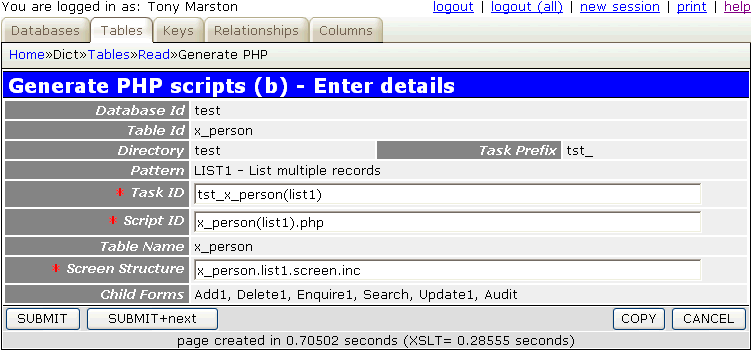When the SUBMIT button is pressed the transaction `tst_x_person(list1)` and its 5 children (add1, delete1, enquire1, update1, search) will be added to the MNU_TASK table, and the children will be added to the navigation bar of `tst_x_person(list1)`. Additionally, `tst_x_person(list1)` will be added to the subsystem's menu as created in the Build Directory stage.

The following component scripts will also be created:

1. x_person(list1).php
```<?php
\$table_id = 'x_person';                      // table name
\$screen   = 'x_person.list1.screen.inc';     // file identifying screen structure
require 'std.list1.inc';                     // activate page controller
?>
```
```<?php
\$table_id = 'x_person';                      // table id
\$screen   = 'x_person.detail.screen.inc';    // file identifying screen structure
require 'std.add1.inc';                      // activate page controller
?>
```
3. x_person(del1).php
```<?php
\$table_id = 'x_person';                      // table id
\$screen   = 'x_person.detail.screen.inc';    // file identifying screen structure
require 'std.delete1.inc';                   // activate page controller
?>
```
4. x_person(enq1).php
```<?php
\$table_id = 'x_person';                      // table id
\$screen   = 'x_person.detail.screen.inc';    // file identifying screen structure
require 'std.enquire1.inc';                  // activate page controller
?>
```
5. x_person(search).php
```<?php
\$table_id = 'x_person';                      // table id
\$screen   = 'x_person.detail.screen.inc';    // file identifying screen structure
require 'std.search1.inc';                   // activate page controller
?>
```
6. x_person(upd1).php
```<?php
\$table_id = 'x_person';                      // table id
\$screen   = 'x_person.detail.screen.inc';    // file identifying screen structure
require 'std.update1.inc';                   // activate page controller
?>
```

The following screen structure scripts will also be created:

1. x_person.list1.screen.inc
```<?php
\$structure['xsl_file'] = 'std.list1.xsl';

\$structure['tables']['main'] = 'x_person';

// identify the column specs - may use 'width' or 'class'
\$structure['main']['columns'][] = array('width' => 5);
\$structure['main']['columns'][] = array('width' => '4.76%');
\$structure['main']['columns'][] = array('width' => '4.76%');
\$structure['main']['columns'][] = array('width' => '4.76%');
\$structure['main']['columns'][] = array('width' => '4.76%');
\$structure['main']['columns'][] = array('width' => '4.76%');
\$structure['main']['columns'][] = array('width' => '4.76%');
\$structure['main']['columns'][] = array('width' => '4.76%');
\$structure['main']['columns'][] = array('width' => '4.76%');
\$structure['main']['columns'][] = array('width' => '4.76%');
\$structure['main']['columns'][] = array('width' => '4.76%');
\$structure['main']['columns'][] = array('width' => '4.76%');
\$structure['main']['columns'][] = array('width' => '4.76%');
\$structure['main']['columns'][] = array('width' => '4.76%');
\$structure['main']['columns'][] = array('width' => '4.76%');
\$structure['main']['columns'][] = array('width' => '4.76%');
\$structure['main']['columns'][] = array('width' => '4.76%');
\$structure['main']['columns'][] = array('width' => '4.76%');
\$structure['main']['columns'][] = array('width' => '4.76%');
\$structure['main']['columns'][] = array('width' => '4.76%');
\$structure['main']['columns'][] = array('width' => '4.76%');
\$structure['main']['columns'][] = array('width' => '4.76%');

// identify the field names and their screen labels
\$structure['main']['fields'][] = array('selectbox' => 'Select');
\$structure['main']['fields'][] = array('person_id' => 'Person Id');
\$structure['main']['fields'][] = array('pers_type_id' => 'Pers Type Id');
\$structure['main']['fields'][] = array('node_id' => 'Node Id');
\$structure['main']['fields'][] = array('nat_ins_no' => 'Nat Ins No');
\$structure['main']['fields'][] = array('first_name' => 'First Name');
\$structure['main']['fields'][] = array('last_name' => 'Last Name');
\$structure['main']['fields'][] = array('initials' => 'Initials');
\$structure['main']['fields'][] = array('star_sign' => 'Star Sign');
\$structure['main']['fields'][] = array('value1' => 'Value1');
\$structure['main']['fields'][] = array('value2' => 'Value2');
\$structure['main']['fields'][] = array('start_date' => 'Start Date');
\$structure['main']['fields'][] = array('end_date' => 'End Date');
\$structure['main']['fields'][] = array('picture' => 'Picture');
\$structure['main']['fields'][] = array('favourite_food' => 'Favourite Food');
\$structure['main']['fields'][] = array('fckeditor_test' => 'Fckeditor Test');
\$structure['main']['fields'][] = array('created_date' => 'Created Date');
\$structure['main']['fields'][] = array('created_user' => 'Created User');
\$structure['main']['fields'][] = array('revised_date' => 'Revised Date');
\$structure['main']['fields'][] = array('revised_user' => 'Revised User');
?>
```
2. x_person.detail.screen.inc
```<?php
\$structure['xsl_file'] = 'std.detail1.xsl';

\$structure['tables']['main'] = 'x_person';

// identify the column specs - may use 'width' or 'class'
\$structure['main']['columns'][] = array('width' => '25%');
\$structure['main']['columns'][] = array('width' => '*');

// identify the contents of each row in the table
\$structure['main']['fields'][] = array('person_id' => 'Person Id');
\$structure['main']['fields'][] = array('pers_type_id' => 'Pers Type Id');
\$structure['main']['fields'][] = array('node_id' => 'Node Id');
\$structure['main']['fields'][] = array('nat_ins_no' => 'Nat Ins No');
\$structure['main']['fields'][] = array('first_name' => 'First Name');
\$structure['main']['fields'][] = array('last_name' => 'Last Name');
\$structure['main']['fields'][] = array('initials' => 'Initials');
\$structure['main']['fields'][] = array('star_sign' => 'Star Sign');
\$structure['main']['fields'][] = array('value1' => 'Value1');
\$structure['main']['fields'][] = array('value2' => 'Value2');
\$structure['main']['fields'][] = array('start_date' => 'Start Date');
\$structure['main']['fields'][] = array('end_date' => 'End Date');
\$structure['main']['fields'][] = array('picture' => 'Picture');
\$structure['main']['fields'][] = array('favourite_food' => 'Favourite Food');
\$structure['main']['fields'][] = array('fckeditor_test' => 'Fckeditor Test');
\$structure['main']['fields'][] = array('created_date' => 'Created Date');
\$structure['main']['fields'][] = array('created_user' => 'Created User');
\$structure['main']['fields'][] = array('revised_date' => 'Revised Date');
\$structure['main']['fields'][] = array('revised_user' => 'Revised User');
?>
```

### 3. Modify the screen structure files

If you run transaction `tst_x_person(list1)` with its default screen structure you will see that it has too many fields and overflows the page width, so its needs to be customised. If you make the amendments show below the result will be as shown in Figure 2.

```<?php
\$structure['xsl_file'] = 'std.list1.xsl';

\$structure['tables']['main'] = 'x_person';

// identify the column specs - may use 'width' or 'class'
\$structure['main']['columns'][] = array('width' => 5);
\$structure['main']['columns'][] = array('width' => 70);
\$structure['main']['columns'][] = array('width' => 100);
\$structure['main']['columns'][] = array('width' => 100);
\$structure['main']['columns'][] = array('width' => 100);
\$structure['main']['columns'][] = array('width' => '*');

// identify the field names and their screen labels
\$structure['main']['fields'][] = array('selectbox' => 'Select');
\$structure['main']['fields'][] = array('person_id' => 'Person Id');
\$structure['main']['fields'][] = array('first_name' => 'First Name');
\$structure['main']['fields'][] = array('last_name' => 'Last Name');
\$structure['main']['fields'][] = array('star_sign' => 'Star Sign');
\$structure['main']['fields'][] = array('pers_type_desc' => 'Person Type');
?>
```

Figure 2 - list X_PERSON screen (modified)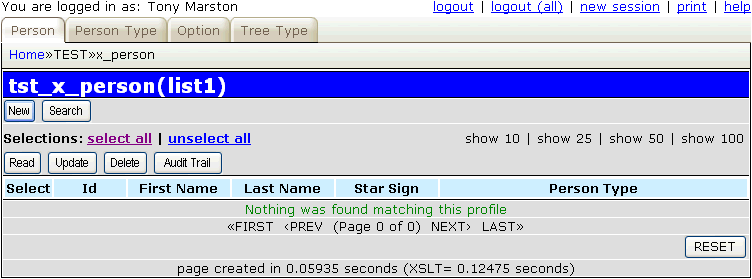If you press the 'New' button to run the Add1 transaction you will see the screen shown in Figure 3:

Figure 3 - add X_PERSON screen (original)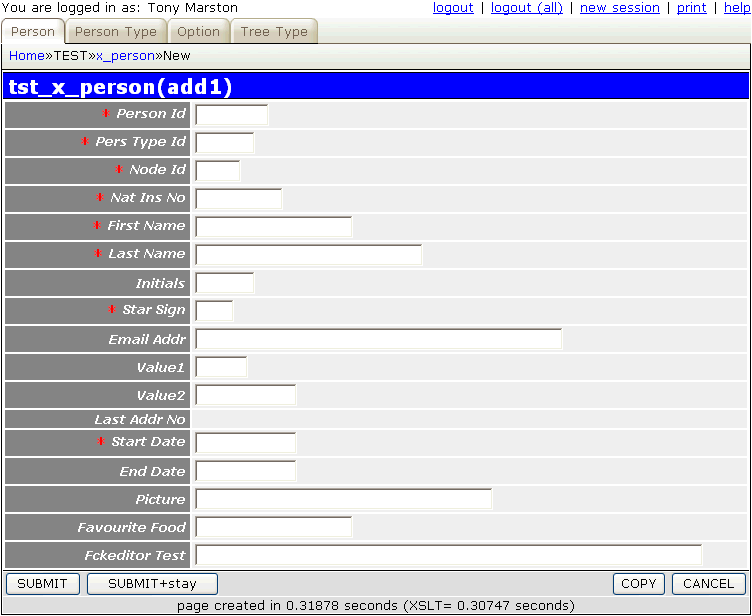It is possible to adjust this layout so that instead of a purely vertical arrangement some of the fields are side-by-side on the same line. If you make the amendments show below the result will be as shown in Figure 4.

```<?php
\$structure['xsl_file'] = 'std.detail1.xsl';

\$structure['tables']['main'] = 'x_person';

// identify the column specs - may use 'width' or 'class'
\$structure['main']['columns'][] = array('width' => '20%');
\$structure['main']['columns'][] = array('width' => '25%');
\$structure['main']['columns'][] = array('width' => '15%');
\$structure['main']['columns'][] = array('width' => '25%');
\$structure['main']['columns'][] = array('width' => '10%');
\$structure['main']['columns'][] = array('width' => '10%');

// identify the field names and their screen labels
\$structure['main']['fields'] = array('person_id' => 'Id', 'colspan' => 5);

\$structure['main']['fields'][] = array('label' => 'First Name');
\$structure['main']['fields'][] = array('field' => 'first_name', 'size' => 15);
\$structure['main']['fields'][] = array('label' => 'Last Name');
\$structure['main']['fields'][] = array('field' => 'last_name', 'size' => 15);
\$structure['main']['fields'][] = array('label' => 'Initials');
\$structure['main']['fields'][] = array('field' => 'initials');

\$structure['main']['fields'] = array('picture' => 'Picture', 'colspan' => 5);
\$structure['main']['fields'] = array('nat_ins_no' => 'Nat. Ins. No.', 'colspan' => 5);
\$structure['main']['fields'] = array('pers_type_id' => 'Person Type', 'colspan' => 5);
\$structure['main']['fields'] = array('star_sign' => 'Star Sign', 'colspan' => 5);
\$structure['main']['fields'] = array('node_id' => 'Organisation', 'colspan' => 5);
\$structure['main']['fields'] = array('email_addr' => 'E-mail', 'colspan' => 5);

\$structure['main']['fields'][] = array('label' => 'Value 1');
\$structure['main']['fields'][] = array('field' => 'value1');
\$structure['main']['fields'][] = array('label' => 'Favourite Food', 'rowspan' => 3);
\$structure['main']['fields'][] = array('field' => 'favourite_food', 'colspan' => 3, 'rowspan' => 3);

// these next two fields fit to the left of 'favourite_food' and must be displayed, even when empty
\$structure['main']['fields'][] = array('label' => 'Value 2');
\$structure['main']['fields'][] = array('field' => 'value2', 'display-empty' => 'y');
\$structure['main']['fields'][] = array('label' => 'Last Address No');
\$structure['main']['fields'][] = array('field' => 'last_addr_no', 'display-empty' => 'y');

\$structure['main']['fields'][] = array('label' => 'Start Date');
\$structure['main']['fields'][] = array('field' => 'start_date');
\$structure['main']['fields'][] = array('label' => 'End Date');
\$structure['main']['fields'][] = array('field' => 'end_date', 'colspan' => 3);

\$structure['main']['fields'][] = array('created_date' => 'Created Date', 'colspan' => 5);
\$structure['main']['fields'][] = array('created_user' => 'Created By', 'colspan' => 5);
\$structure['main']['fields'][] = array('revised_date' => 'Revised Date', 'colspan' => 5);
\$structure['main']['fields'][] = array('revised_user' => 'Revised By', 'colspan' => 5);
?>
```

Figure 4 - add X_PERSON screen (modified)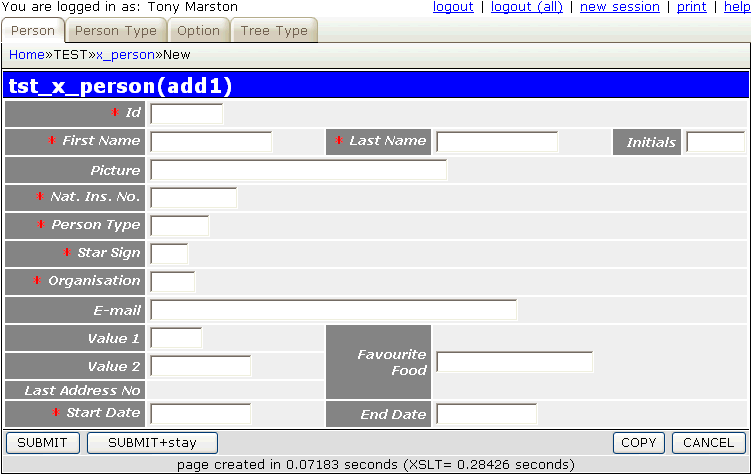This screen only allows text input for each field, so some alterations are required in order to convert some to use dropdown lists and file pickers.

### 4. Modify screen labels and button text

Follow the procedure described in Part 2 of this tutorial to replace the default text with customised values.

As the number of possible star signs is strictly limited (there are only 12) it would be better to allow the user to pick from a list instead of typing into a text box. This is what a dropdown list is for - it presents the user with a list of options and allows him to choose one. The first step is to modify the data dictionary for this table using the values shown below:

Table Column Setting Value
x_person star_sign control DROPDOWN LIST
option_list star_sign

For a description of what these settings mean please refer to Update Column.

After completing this change you must run the Export to PHP function in order to update the `x_person.dict.inc` file, otherwise the application will not know that anything has changed. This will create the following entry in `x_person.dict.inc`:

```    \$fieldspec['star_sign'] = array('type' => 'string',
'size' => 3,
'required' => 'y',
'control' => 'dropdown',
'optionlist' => 'star_sign');
```

The following code is needed in the _cm_getExtraData() method of the `x_person.class.inc` file in order to obtain the list of values and load it into the object's `lookup_data` variable that will be exported to the `<lookup>` element in the XML document. From here it will be loaded into the HTML output during the XSL transformation.

```    function _cm_getExtraData (\$where, \$fieldarray)
// Perform custom processing for the getExtraData method.
// \$where = a string in SQL 'where' format.
// \$fieldarray = the contents of \$where as an array.
{
// get values for star_sign and insert into lookup array
\$array = \$this->getValRep('star_sign');
\$this->lookup_data['star_sign'] = \$array;

return \$fieldarray;

```

The following code is needed in the _cm_getValRep() method of the `x_person.class.inc` file in order to provide the list of values:

```    function _cm_getValRep (\$item, \$where)
// get Value/Representation list as an associative array.
{
\$array = array();

if (\$item == 'star_sign') {
\$array = getLanguageArray('star_sign');
return \$array;
} // if

return \$array;

} // _cm_getValRep
```

Note the use of the getLanguageArray() function instead of having hard-coded text. This requires an entry in the `text/<language>/language_array.inc` file as follows:

```\$array['star_sign'] = array('ARI' => 'Aries',
'AQU' => 'Aquarius',
'CAN' => 'Cancer',
'CAP' => 'Capricorn',
'GEM' => 'Gemini',
'LEO' => 'Leo',
'LIB' => 'Libra',
'PIS' => 'Pisces',
'SAG' => 'Sagittarius',
'SCO' => 'Scorpio',
'TAU' => 'Taurus',
'VIR' => 'Virgo');
```

If your application is required to support more than one language all you need do is to translate that text and place it in the `language_array.inc` file in the relevant `<language>` subdirectory.

When this data is exported to the XML document it will look like the following:

```<x_person>
.....
<star_sign size="3" required="y" control="dropdown" optionlist="star_sign" />
.....
</x_person>
<lookup>
<star_sign>
<option id="" />
<option id="ARI">Aries</option>
<option id="AQU">Aquarius</option>
<option id="CAN">Cancer</option>
<option id="CAP">Capricorn</option>
<option id="GEM">Gemini</option>
<option id="LEO">Leo</option>
<option id="LIB">Libra</option>
<option id="PIS">Pisces</option>
<option id="SAG">Sagittarius</option>
<option id="SCO">Scorpio</option>
<option id="TAU">Taurus</option>
<option id="VIR">Virgo</option>
</star_sign>
</lookup>
```

When this data is written to the HTML output it will look like the following:

```    <tr>
<td class="label">
<span class="required">* </span>Star Sign</td>
<td>
<select class="dropdown" name="star_sign">
<option value="" selected="selected"> </option>
<option value="ARI">Aries</option>
<option value="AQU">Aquarius</option>
<option value="CAN">Cancer</option>
<option value="CAP">Capricorn</option>
<option value="GEM">Gemini</option>
<option value="LEO">Leo</option>
<option value="LIB">Libra</option>
<option value="PIS">Pisces</option>
<option value="SAG">Sagittarius</option>
<option value="SCO">Scorpio</option>
<option value="TAU">Taurus</option>
<option value="VIR">Virgo</option>
</select>
</td>
</tr>
```

### 6. Convert PERS_TYPE_ID into a dropdown list

This is similar to the procedure for STAR_SIGN, but with some sight differences. The first step is to modify the data dictionary for this table using the values shown below:

Table Column Setting Value
x_person pers_type_id control DROPDOWN LIST
option_list pers_type_id

For a description of what these settings mean please refer to Update Column.

After completing this change you must run the Export to PHP function in order to update the `x_person.dict.inc` file, otherwise the application will not know that anything has changed. This will create the following entry in `x_person.dict.inc`:

```\$fieldspec['pers_type_id'] = array('type' => 'string',
'size' => 6,
'required' => 'y',
'control' => 'dropdown',
'optionlist' => 'pers_type_id');
```

The contents of this list does not come from a static source but from the contents of another database table, so we must access that database table in order to obtain the current contents. The following code is needed in the _cm_getExtraData() method of the `x_person.class.inc` file in order to obtain the list of values and load it into the object's `lookup_data` variable.

```    function _cm_getExtraData (\$where, \$fieldarray)
// Perform custom processing for the getExtraData method.
// \$where = a string in SQL 'where' format.
// \$fieldarray = the contents of \$where as an array.
{
// get values for star_sign and insert into lookup array
\$array = \$this->getValRep('star_sign');
\$this->lookup_data['star_sign'] = \$array;

// get contents of foreign table PERS_TYPE and add to lookup array
\$dbobject = RDCsingleton::getInstance('x_pers_type');
\$array = \$dbobject->getValRep('pers_type_id');
\$this->lookup_data['pers_type_id'] = \$array;

return \$fieldarray;

```

The following code is needed in the _cm_getValRep() method of the `x_pers_type.class.inc` file in order to provide the list of values:

```    function _cm_getValRep (\$item=null, \$where=null)
// get Value/Representation list as an associative array.
{
\$array = array();

if (\$item == 'pers_type_id') {
// get data from the database
\$this->sql_select     = 'pers_type_id, pers_type_desc';
\$this->sql_orderby    = 'pers_type_id';
\$this->sql_ordery_seq = 'asc';
\$data = \$this->getData(\$where);

// convert each row into 'id=desc' in the output array
foreach (\$data as \$row => \$rowdata) {
\$rowvalues = array_values(\$rowdata);
\$array[\$rowvalues] = \$rowvalues;
} // foreach

return \$array;
} // if

return \$array;

} // _cm_getValRep
```

### 7. Convert FAVOURITE_FOOD into a multi-dropdown list

Unlike an ordinary dropdown list which can only allow a single selection, this type of control will allow multiple selections to be made. In order for this to work the database schema must allow multiple selections to be stored. In MySQL this can be done as follows:

```  `favourite_food` set('1','2','3','4','5','6','7','8','9','10') default NULL,
```

In PostgreSQL this can be done by using the `array` type as follows:

```  favourite_food varchar(2)[],
```

Note that the MySQL version defines all the possible values that may be selected whereas the PostgreSQL version simply defines an array of `varchar(2)` elements without explicitly stating what the allowable values are or placing a limit on their number.

The first step is to modify the data dictionary for this table using the values shown below:

Table Column Setting Value
x_person favourite_food control MULTI-DROPDOWN
option_list favourite_food

For a description of what these settings mean please refer to Update Column.

After completing this change you must run the Export to PHP function in order to update the `x_person.dict.inc` file, otherwise the application will not know that anything has changed. This will create the following entry in `x_person.dict.inc`:

```\$fieldspec['favourite_food'] = array('type' => 'set',
'values' => array('1', '2', '3', '4', '5', '6', '7', '8', '9', '10'),
'control' => 'multidrop',
'optionlist' => 'favourite_food');
```

The following code is needed in the _cm_getExtraData() method of the `x_person.class.inc` file in order to obtain the list of values:

```    function _cm_getExtraData (\$where, \$fieldarray)
// Perform custom processing for the getExtraData method.
// \$where = a string in SQL 'where' format.
// \$fieldarray = the contents of \$where as an array.
{
// get values for star_sign and insert into lookup array
\$array = \$this->getValRep('star_sign');
\$this->lookup_data['star_sign'] = \$array;

// get values for favourite_food and insert into lookup array
\$array = \$this->getValRep('favourite_food');
\$this->lookup_data['favourite_food'] = \$array;

// get contents of foreign table PERS_TYPE and add to lookup array
\$dbobject = RDCsingleton::getInstance('x_pers_type');
\$array = \$dbobject->getValRep('pers_type_id');
\$this->lookup_data['pers_type_id'] = \$array;

return \$fieldarray;

```

The following code is needed in the _cm_getValRep() method of the `x_pers_type.class.inc` file in order to provide the list of values:

```    function _cm_getValRep (\$item='', \$where)
// get Value/Representation list as an associative array.
{
\$array = array();

if (\$item == 'star_sign') {
\$array = getLanguageArray('star_sign');
return \$array;
} // if

if (\$item == 'favourite_food') {
\$array = getLanguageArray('favourite_food');
return \$array;
} // if

return \$array;

} // _cm_getValRep
```

As the list of entries is static it requires an entry in the `text/<language>/language_array.inc` file as follows:

```\$array['favourite_food'] = array('1' => 'Eggs',
'2' => 'Bacon',
'3' => 'Chips',
'4' => 'Beans',
'5' => 'Sausages',
'6' => 'Mushrooms',
'7' => 'Tomatoes',
'8' => 'Hash Browns',
'9' => 'Toast',
```

### 8. Convert NODE_ID into a popup

If the list of options in a dropdown list is too large then this type of control could be used instead as it activates another form. This allows the user to browse through the database table which contains the options, set selection criteria, and even to create new entries to add to the list.

The first step is to create the POPUP form to be used, which will be based on the List Tree Structure screen created previously. Using the Generate Transactions procedure select the X_TREE_NODE table, the POPUP4 pattern, then press the SUBMIT button to bring up the screen shown in Figure 5:

Figure 5 - create POPUP transaction for the X_TREE_NODE table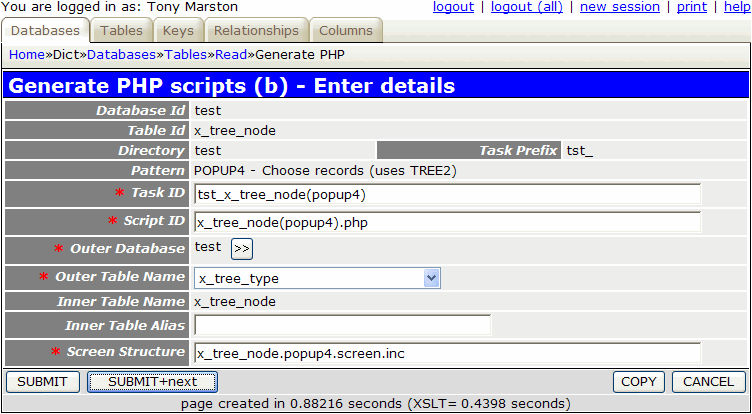When you press the SUBMIT button this will create the following:

It would be a good idea to amend the contents of `x_tree_node.popup4.screen.inc` so that it resembles the previously customised x_tree_node.tree2.screen.inc file.

The second step is to modify the data dictionary for this table using the values shown below:

Table Column Setting Value
x_person node_id control POPUP
Foreign Field node_desc

For a description of what these settings mean please refer to Update Column.

After completing this change you must run the Export to PHP function in order to update the `x_person.dict.inc` file, otherwise the application will not know that anything has changed. This will create the following entry in `x_person.dict.inc`:

```\$fieldspec['node_id'] = array('type' => 'integer',
'size' => 4,
'minvalue' => 0,
'maxvalue' => 65535,
'required' => 'y',
'default' => '0',
'control' => 'popup',
'foreign_field' => 'node_desc');
```

This will need an entry from the X_TREE_TYPE table to be pre-selected before it can function, so the following code needs to be inserted into the _cm_popupCall() method of the `x_person.class.inc` file.

```   function _cm_popupCall (\$popupname, \$where, \$fieldarray, &\$settings)
// if a popup button has been pressed the contents of \$where amy need to
// be altered before the popup screen is called.
// NOTE: \$settings is passed BY REFERENCE as it may be altered.
{
if (\$popupname == 'tst_x_tree_node(popup4)') {
// structure must be of type 'ORG'
\$where = "tree_type_id='ORG'";
} // if

// allow only one entry to be selected (the default)
\$settings['select_one'] = TRUE;

return \$where;

} // _cm_popupCall
```

### 9. Convert PICTURE into a file picker

This is similar to a popup, but instead of picking an entry from the database it allows the user to pick a file from the file system, and it is the filename which is then stored in the database.

The first step is to create the FILE PICKER form to be used. Using the Generate Transactions procedure select the X_PERSON table, the FILEPICKER pattern, then press the SUBMIT button to bring up the screen shown in Figure 6:

Figure 6 - create FILE PICKER transaction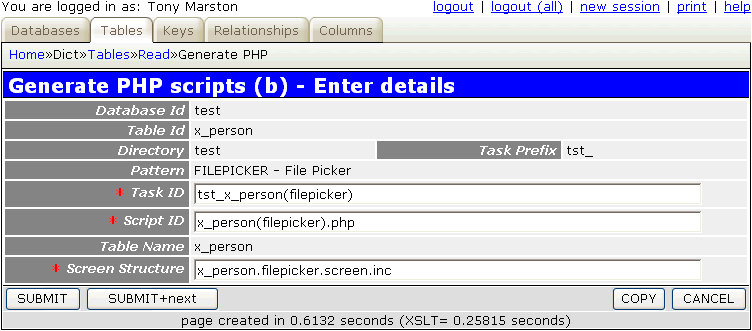When you press the SUBMIT button this will create the following:

The following component script will be created:

```<?php
\$table_id = 'x_person';                       // table id
\$screen   = 'x_person.filepicker.screen.inc'; // file identifying screen structure
require 'std.filepicker1.inc';                // activate page controller
?>
```

The following code is needed in the _cm_initialiseFilePicker() method of file `x_person.class.inc` in order to identify the subdirectory which contains the files to be displayed, as well as the types of files to be displayed. Any file with a type which is not in this list will be excluded.

```    function _cm_initialiseFilePicker ()
// perform any initialisation before displaying the File Picker screen.
{
// identify the subdirectory which contains the files
\$this->picker_subdir    = 'pictures';

// identify the file types that may be picked
\$this->picker_filetypes = array('bmp', 'jpg', 'png', 'gif');

return;

} // _cm_initialiseFilePicker
```

You will need to ensure that this subdirectory exists and contains files that can be picked.

You can set the size of the thumbnail image to be displayed by modifying the screen structure script, as shown below.

```<?php
\$structure['xsl_file'] = 'std.filepicker.list1.xsl';

\$structure['tables']['main'] = 'file';

\$structure['main']['columns'][] = array('width' => '40%');
\$structure['main']['columns'][] = array('width' => '10%');

\$structure['main']['fields'][] = array('file' => 'File Name');
\$structure['main']['fields'][] = array('image' => 'Image',
'imagewidth' => 75,
'imageheight' => 95);

?>
```

Note that the data will be displayed in two column groups, not one, which is why the width of each group totals to 50%.

The next step is to modify the data dictionary for this table using the values shown below:

Table Column Setting Value
x_person picture control FILE PICKER
Subtype IMAGE
Image width 75
Image Height 95

For a description of what these settings mean please refer to Update Column.

After completing this change you must run the Export to PHP function in order to update the x_person.dict.inc file, otherwise the application will not know that anything has changed. This will create the following entry in x_person.dict.inc:

```\$fieldspec['picture'] = array('type' => 'string',
'size' => 40,
'subtype' => 'image',
'imagewidth' => 75,
'imageheight' => 95,
'control' => 'filepicker',
```

As a result of these modifications the screen changes from what is shown in Figure 4 to what is shown in Figure 7:

Figure 7 - add X_PERSON screen (modified)### 10. Custom code for date validation

Although the standard code within the framework will ensure that the `start_date` and `end_date` fields both contain valid dates, some extra validation is required to check that `start_date` is earlier than `end_date`. This can be achieved by inserting the following code into the _cm_commonValidation() method of the `x_person.class.inc` file.

```    function _cm_commonValidation (\$fieldarray, \$orignaldata)
// perform validation that is common to INSERT and UPDATE.
{
if (\$fieldarray['start_date'] > \$fieldarray['end_date']) {
// 'Start Date cannot be later than End Date';
\$this->errors['start_date'] = getLanguageText('e0001');
// 'End Date cannot be earlier than Start Date';
\$this->errors['end_date']   = getLanguageText('e0002');
} // if

return \$fieldarray;

} // _cm_commonValidation
```

• If either of the dates is invalid control will never reach this point - the standard validation class within the framework will issue the appropriate error message(s) and go back to the user.
• During the validation process the date values are converted from user format to database format, so "6 Nov 2006" will appear as "2006-11-06", which makes any date comparisons very easy.
• The `end_date` field is optional, so the user may leave it blank. This would normally cause a problem with date comparisons, but the INFINITY IS NULL setting in the data dictionary will ensure that a null value is converted to infinity (9999-12-31) on input, and converted from infinity back to null on output.

Note also the use of the getLanguageText() function instead of having hard-coded text. This requires entries to be added to the `text/<language>/language_text.inc` file as follows:

```\$array['e0001'] = "Start Date cannot be later than End Date";
\$array['e0002'] = "End Date cannot be earlier than Start Date";
```

### 11. Create data for X_PERSON

You may now proceed to enter a selection of test data, perhaps using a PDF export from the `xample` database as a reference.

## Create transactions for the X_PERSON_ADDR table

Here are the steps necessary to build the components to maintain the X_PERSON_ADDR table within the test database.

### 1. Modify Data Dictionary for X_PERS_ADDR table

The first step is to modify the data dictionary for this table using the values shown below:

Table Column Setting
town UPPERCASE
end_date INFINITY IS NULL
created_date NOEDIT, AUTO-INSERT, NOSEARCH
created_user NOEDIT, AUTO-INSERT, NOSEARCH
revised_date NOEDIT, AUTO-UPDATE, NOSEARCH
revised_user NOEDIT, AUTO-UPDATE, NOSEARCH

For a description of what these settings mean please refer to Update Column.

It would also be useful to specify custom validation for this table using the values shown below:

Table Column Custom Validation
fax_no sample.validation.class.inc/sample_validation_class/fax_no
postcode sample.validation.class.inc/sample_validation_class/postcode

This is explained in RADICORE for PHP - Extending the Validation class.

After these changes have been made they must be made available to the application by running the Export to PHP function.

### 2. Generate LIST2 Transaction

This transaction is going to be accessed from a navigation button within the List X_PERSON transaction, not directly from a menu button, therefore we need to use the LIST2 pattern. Using the Generate Transactions procedure select the X_PERSON_ADDR table, the LIST2 pattern, then press the SUBMIT button to bring up the screen shown in Figure 8:

Figure 8 - create LIST2 transaction for the X_PERSON_ADDR table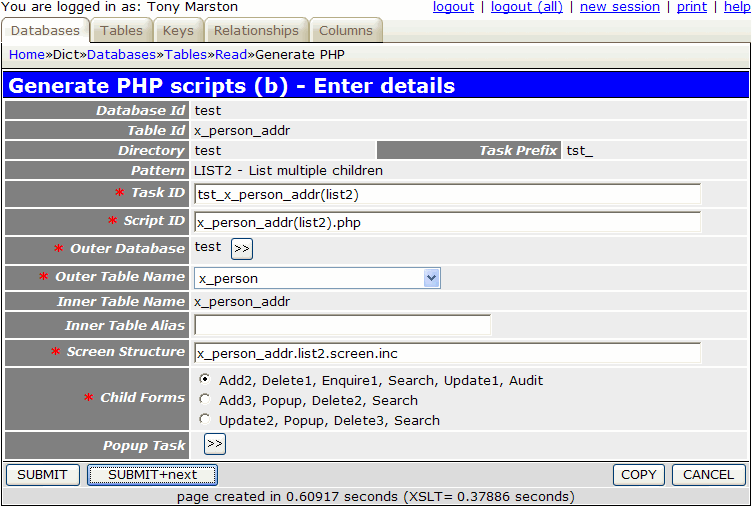When the SUBMIT button is pressed the transaction `tst_x_person_addr(list2)` and its 5 children (add2, delete1, enquire1, update1, search) will be added to the MNU_TASK table.

The following component scripts will also be created:

```<?php
\$outer_table = 'x_person';                          // name of outer (parent) table
\$inner_table = 'x_person_addr';                     // name of inner (child) table
\$screen      = 'x_person_addr.list2.screen.inc';    // file identifying screen structure
require 'std.list2.inc';                            // activate page controller
?>
```
```<?php
\$table_id = 'x_person_addr';                      // table name
\$screen   = 'x_person_addr.detail.screen.inc';    // file identifying screen structure
require 'std.add2.inc';                           // activate page controller
?>
```
```<?php
\$table_id = 'x_person_addr';                      // table name
\$screen   = 'x_person_addr.detail.screen.inc';    // file identifying screen structure
require 'std.del1.inc';                           // activate page controller
?>
```
```<?php
\$table_id = 'x_person_addr';                      // table name
\$screen   = 'x_person_addr.detail.screen.inc';    // file identifying screen structure
require 'std.enq1.inc';                           // activate page controller
?>
```
```<?php
\$table_id = 'x_person_addr';                      // table name
\$screen   = 'x_person_addr.detail.screen.inc';    // file identifying screen structure
require 'std.search.inc';                         // activate page controller
?>
```
```<?php
\$table_id = 'x_person_addr';                      // table name
\$screen   = 'x_person_addr.detail.screen.inc';    // file identifying screen structure
require 'std.upd1.inc';                           // activate page controller
?>
```

The following screen structure scripts will also be created:

```<?php
\$structure['xsl_file'] = 'std.list2.xsl';

\$structure['tables']['outer'] = 'x_person';

// identify the column specs - may use 'width' or 'class'
\$structure['outer']['columns'][] = array('width' => '25%');
\$structure['outer']['columns'][] = array('width' => '*');

// identify the field names and their screen labels
\$structure['outer']['fields'][] = array('person_id' => 'Person Id');
\$structure['outer']['fields'][] = array('pers_type_id' => 'Pers Type Id');
\$structure['outer']['fields'][] = array('node_id' => 'Node Id');
\$structure['outer']['fields'][] = array('nat_ins_no' => 'Nat Ins No');
\$structure['outer']['fields'][] = array('first_name' => 'First Name');
\$structure['outer']['fields'][] = array('last_name' => 'Last Name');
\$structure['outer']['fields'][] = array('initials' => 'Initials');
\$structure['outer']['fields'][] = array('star_sign' => 'Star Sign');
\$structure['outer']['fields'][] = array('value1' => 'Value1');
\$structure['outer']['fields'][] = array('value2' => 'Value2');
\$structure['outer']['fields'][] = array('start_date' => 'Start Date');
\$structure['outer']['fields'][] = array('end_date' => 'End Date');
\$structure['outer']['fields'][] = array('picture' => 'Picture');
\$structure['outer']['fields'][] = array('favourite_food' => 'Favourite Food');
\$structure['outer']['fields'][] = array('fckeditor_test' => 'Fckeditor Test');
\$structure['outer']['fields'][] = array('created_date' => 'Created Date');
\$structure['outer']['fields'][] = array('created_user' => 'Created User');
\$structure['outer']['fields'][] = array('revised_date' => 'Revised Date');
\$structure['outer']['fields'][] = array('revised_user' => 'Revised User');

// identify the column specs - may use 'width' or 'class'
\$structure['inner']['columns'][] = array('width' => 5);
\$structure['inner']['columns'][] = array('width' => '6.25%');
\$structure['inner']['columns'][] = array('width' => '6.25%');
\$structure['inner']['columns'][] = array('width' => '6.25%');
\$structure['inner']['columns'][] = array('width' => '6.25%');
\$structure['inner']['columns'][] = array('width' => '6.25%');
\$structure['inner']['columns'][] = array('width' => '6.25%');
\$structure['inner']['columns'][] = array('width' => '6.25%');
\$structure['inner']['columns'][] = array('width' => '6.25%');
\$structure['inner']['columns'][] = array('width' => '6.25%');
\$structure['inner']['columns'][] = array('width' => '6.25%');
\$structure['inner']['columns'][] = array('width' => '6.25%');
\$structure['inner']['columns'][] = array('width' => '6.25%');
\$structure['inner']['columns'][] = array('width' => '6.25%');
\$structure['inner']['columns'][] = array('width' => '6.25%');
\$structure['inner']['columns'][] = array('width' => '6.25%');
\$structure['inner']['columns'][] = array('width' => '6.25%');

// identify the field names and their screen labels
\$structure['inner']['fields'][] = array('selectbox' => 'Select');
\$structure['inner']['fields'][] = array('person_id' => 'Person Id');
\$structure['inner']['fields'][] = array('telephone_no' => 'Telephone No');
\$structure['inner']['fields'][] = array('fax_no' => 'Fax No');
\$structure['inner']['fields'][] = array('town' => 'Town');
\$structure['inner']['fields'][] = array('county' => 'County');
\$structure['inner']['fields'][] = array('postcode' => 'Postcode');
\$structure['inner']['fields'][] = array('start_date' => 'Start Date');
\$structure['inner']['fields'][] = array('end_date' => 'End Date');
\$structure['inner']['fields'][] = array('created_date' => 'Created Date');
\$structure['inner']['fields'][] = array('created_user' => 'Created User');
\$structure['inner']['fields'][] = array('revised_date' => 'Revised Date');
\$structure['inner']['fields'][] = array('revised_user' => 'Revised User');
?>
```
```<?php
\$structure['xsl_file'] = 'std.detail1.xsl';

// identify the column specs - may use 'width' or 'class'
\$structure['main']['columns'][] = array('width' => '25%');
\$structure['main']['columns'][] = array('width' => '*');

// identify the contents of each row in the table
\$structure['main']['fields'][] = array('person_id' => 'Person Id');
\$structure['main']['fields'][] = array('telephone_no' => 'Telephone No');
\$structure['main']['fields'][] = array('fax_no' => 'Fax No');
\$structure['main']['fields'][] = array('town' => 'Town');
\$structure['main']['fields'][] = array('county' => 'County');
\$structure['main']['fields'][] = array('postcode' => 'Postcode');
\$structure['main']['fields'][] = array('start_date' => 'Start Date');
\$structure['main']['fields'][] = array('end_date' => 'End Date');
\$structure['main']['fields'][] = array('created_date' => 'Created Date');
\$structure['main']['fields'][] = array('created_user' => 'Created User');
\$structure['main']['fields'][] = array('revised_date' => 'Revised Date');
\$structure['main']['fields'][] = array('revised_user' => 'Revised User');
?>
```

This part is not done automatically, so it has to be done manually. By following this procedure you must locate the task `tst_x_person(list1)` and add `tst_x_person_addr(list2)` to its list of navigation buttons.

### 4. Modify the screen structure files

If you run transaction `tst_x_person_addr(list2)` with its default screen structure you will see that it has too many fields and overflows the page width, so its needs to be customised. If you make the amendments show below the result will be as shown in Figure 9.

```<?php
\$structure['xsl_file'] = 'std.list2.xsl';

\$structure['tables']['outer'] = 'x_person';

\$structure['outer']['columns'][] = array('width' => '25%');
\$structure['outer']['columns'][] = array('width' => '5%');
\$structure['outer']['columns'][] = array('width' => '*');

\$structure['outer']['fields'][] = array('label' => 'Person');
\$structure['outer']['fields'][] = array('field' => 'first_name');
\$structure['outer']['fields'][] = array('field' => 'last_name');

\$structure['inner']['columns'][] = array('width' => 5);
\$structure['inner']['columns'][] = array('width' => 20);
\$structure['inner']['columns'][] = array('width' => '*');
\$structure['inner']['columns'][] = array('width' => 150);
\$structure['inner']['columns'][] = array('width' => 100);
\$structure['inner']['columns'][] = array('width' => 100);

\$structure['inner']['fields'][] = array('selectbox' => 'Select');
\$structure['inner']['fields'][] = array('town' => 'Town');
\$structure['inner']['fields'][] = array('county' => 'County');
\$structure['inner']['fields'][] = array('postcode' => 'Postcode');
\$structure['inner']['fields'][] = array('start_date' => 'Start Date');
?>
```

Figure 9 - list X_PERSON_ADDR screen (modified)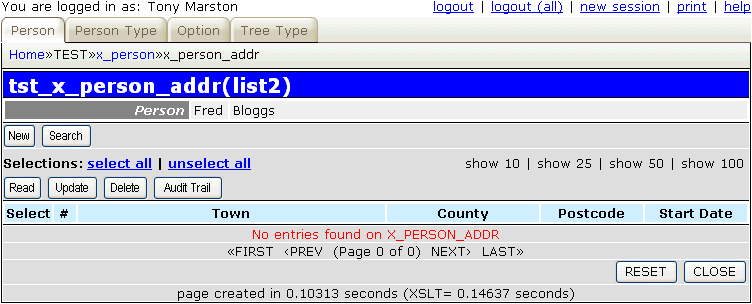If you modify the default screen structure for the detail screen you will see the result shown in Figure 10.

```<?php
\$structure['xsl_file'] = 'std.detail1.xsl';

\$structure['main']['columns'][] = array('width' => '25%');
\$structure['main']['columns'][] = array('width' => '*');

\$structure['main']['fields'][] = array('person_name' => 'Name');
\$structure['main']['fields'][] = array('town' => 'Town');
\$structure['main']['fields'][] = array('county' => 'County');
\$structure['main']['fields'][] = array('postcode' => 'Postcode');
\$structure['main']['fields'][] = array('telephone_no' => 'Telephone No');
\$structure['main']['fields'][] = array('fax_no' => 'Fax No');
\$structure['main']['fields'][] = array('start_date' => 'Start Date');
\$structure['main']['fields'][] = array('end_date' => 'End Date');
\$structure['main']['fields'][] = array('created_date' => 'Created Date');
\$structure['main']['fields'][] = array('created_user' => 'Created User');
\$structure['main']['fields'][] = array('revised_date' => 'Revised Date');
\$structure['main']['fields'][] = array('revised_user' => 'Revised User');
?>
```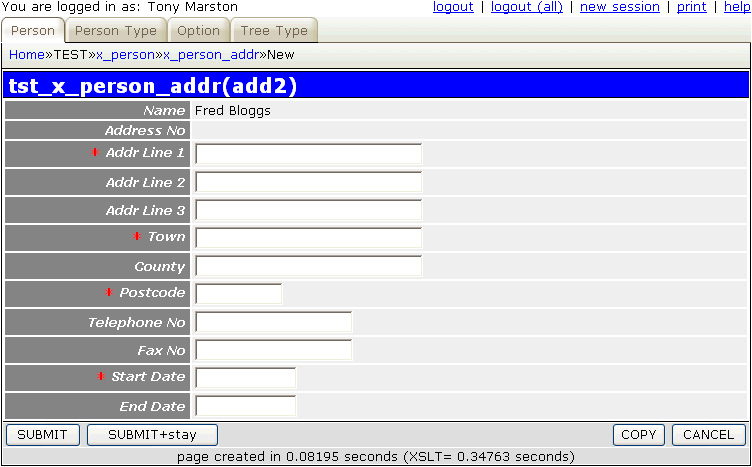You should notice that this refers to a field called `person_name` which does not actually exist in the database schema, so where does it come from? If you look in file `x_person_addr.dict.inc` you will see the following entry:

```\$this->parent_relations[] = array('parent' => 'x_person',
'parent_field' => 'CONCAT(first_name, \' \', last_name) AS person_name',
'fields' => array('person_id' => 'person_id'));
```

This is possible due to the relationship details which exist in the Data Dictionary as explained here. This information is used by the framework to retrieve the specified field(s) from the parent record(s) and add them into the data array for the current record.

### 5. Modify screen labels and button text

Follow the procedure described in Part 2 of this tutorial to replace the default text with customised values.

### 6. Define the business rules

The business rules for person addresses is quite involved and therefore will require some custom code to be added to both the X_PERSON_ADDR and X_PERSON classes. These rules are as follows:

1. The primary key for each X_PERSON_ADDR record is a combination of the following:
• `person_id` which is also the foreign key linking to the X_PERSON table.
• `address_no` which is the address sequence number starting at 1.

This means that the first address for each person is number 1, the second address is number 2, and so on, in strict sequence.

The value for `address_no` is obtained by reading the `last_addr_no` field on the parent X_PERSON record and incrementing it by one. This means the following:

• The primary key for the first address is always `person_id, 1`.
• The primary key for the last address is always `person_id, last_addr_no`.
• The primary key for the previous address is always `person_id, address_no -1`.
• The primary key for the next address is always `person_id, address_no +1`.

This simple arrangement means that any primary key can be constructed without having to search for the address with the earliest/latest or previous/next start date.

2. Each X_PERSON record has a `start_date` and an `end_date`, with the rule being that `end_date` cannot be earlier than `start_date`.
3. Each X_PERSON_ADDR record also has a `start_date` and an `end_date`, with the rule being that `end_date` cannot be earlier than `start_date`.
4. In addition the `start_date` of the first address must be the same as the X_PERSON `start date`, and the `end_date` of the last address must be the same as the X_PERSON `end date`. This ensures that the time period covered on the X_PERSON record is exactly the same as the time period on the associated X_PERSON_ADDR records.
5. The dates on all address records for a person must be contiguous, i.e. they must flow in a continuous and unbroken sequence. No overlaps are allowed, and no missing dates are allowed.
6. If the `start_date` on the X_PERSON record is changed it will also change the `start_date` on the first address record. This date cannot be changed to a value which is greater than the `end_date` of either the X_PERSON record or the first X_PERSON_ADDR record.
7. If the `end_date` on the X_PERSON record is changed it will also change the `end_date` on the last address record. This date cannot be changed to a value which is earlier than the `start_date` of either the X_PERSON record or the last X_PERSON_ADDR record.
8. When inserting address records the following rules apply:
1. The value for `address_no` will be obtained by incrementing the current value of `last_addr_no` on the X_PERSON record.
2. If this is the first address then `start_date` will automatically be set to `start_date` on the X_PERSON record and no changes will be allowed.
3. If this is not the first address then an initial value for `start_date` will be displayed as the `start_date` of the previous address plus 1 day. This may be changed to a later date, but cannot be earlier.
4. When the record is added to the database and there is a previous address then the `end_date` of the previous address will be updated to the `start_date` of the current address minus 1 day.
5. The `end_date` will be set to `end_date` on the X_PERSON record and no changes will be allowed.
9. When updating address records the following rules apply:
1. It is not possible to change the `start_date` of the first address record. This can only be done via the Update Person screen.
2. If there is a previous address then the `start_date` of the current address must be later than the `start_date` of the previous address.
3. When the record is written to the database and there is a previous address then the `end_date` of the previous address will be updated to the `start_date` of the current address minus 1 day.
4. It is not possible to change the `end_date` of the last address record. This can only be done via the Update Person screen.
5. If there is a next address then the `end_date` of the current address must be earlier than the `end_date` of the next address.
6. When the record is written to the database and there is a next address then the `start_date` of the next address will be updated to the `end_date` of the current address plus 1 day.
10. When deleting address records the following rules apply:
1. In order to ensure that address sequence numbers start at 1 and continue in an unbroken sequence it is not possible to delete any address record for a person other than the last address.
2. When the last address is deleted and a previous address exists then the `end_date` of the previous address must be set to the `end_date` of the record being deleted.
3. When the last address is deleted the value in `last_addr_no` on the X_PERSON record must be set to the `address_no` of the deleted record minus 1.

### 7. Modify LANGUAGE_TEXT.INC file for the error messages

The following error messages should first be placed in the `text/en/language_text.inc` file so that they can be accessed by the getLanguageText() method.

```\$array['e0001'] = "Start Date cannot be later than End Date";
\$array['e0002'] = "End Date cannot be earlier than Start Date";
\$array['e0003'] = "This is the lowest level - no children allowed";
\$array['e0004'] = "Cannot be empty";
\$array['e0005'] = "Value too large (%1\\$s)";
\$array['e0006'] = "Must be an integer";
\$array['e0007'] = "Must be greater than 0";
\$array['e0008'] = "Must not be greater than %1\\$s";
\$array['e0009'] = "Value has already been used";

\$array['e0010'] = "Could not locate first PERS_ADDR record";
\$array['e0011'] = "Start Date cannot be later than End Date of first address";
\$array['e0012'] = "Could not locate last PERS_ADDR record";
\$array['e0013'] = "End Date cannot be earlier than Start Date of last address";
\$array['e0014'] = "Could not locate next PERS_ADDR record";
\$array['e0015'] = "End Date must be earlier than End Date of next entry";
\$array['e0016'] = "Could not locate previous PERS_ADDR record";
\$array['e0017'] = "Start Date must be later than Start Date of previous entry";
\$array['e0018'] = "Cannot change start date of first address.";
\$array['e0019'] = "Cannot change end date of last address.";

\$array['e0020'] = "";
\$array['e0021'] = "";
\$array['e0022'] = "";
\$array['e0023'] = "Could not locate PERSON record.";
\$array['e0024'] = "You have not selected an address for deletion.";
\$array['e0025'] = "Only the last address can be deleted.";

// these entries are used by 'sample.validation.class.inc'
\$array['sample001'] = "Invalid format for a postcode.";
\$array['sample002'] = "Invalid format for a telephone number.";
\$array['sample003'] = "Invalid format for a fax number.";
```

Translations in other languages can be placed in the relevant language subdirectories.

The following code goes into the _cm_getInitialData() method:

```    function _cm_getInitialData (\$fieldarray)
// Perform custom processing for the getInitialData method.
// \$fieldarray contains data from the initial \$where clause.
{
\$dbobject = RDCsingleton::getInstance('x_person');

\$where = array2where(\$fieldarray, \$dbobject->getPkeyNames());
\$person_data = \$dbobject->getData_raw(\$where);
if (\$dbobject->numrows <> 1) {
// 'Could not locate PERSON record'
\$this->errors[] = getLanguageText('e0023');
return \$fieldarray;
} // if

\$person_data = \$person_data;  // use data from first row only

// this field is generated, not input by the user

// end_date must be same as person.end_date (and not editable)
\$fieldarray['end_date'] = \$person_data['end_date'];    <-- Rule 8e
\$this->fieldspec['end_date']['noedit'] = 'y';

// for 1st address start_date must be same as person.start_date (and not editable)
\$fieldarray['start_date'] = \$person_data['start_date'];    <-- Rule 8b
\$this->fieldspec['start_date']['noedit'] = 'y';
} else {
if (empty(\$fieldarray['start_date'])) {
// for subsequent addresses the start date must be later than
// the start date of the previous address
\$this->sql_select = 'start_date,end_date';    <-- Rule 8c
\$where_array['person_id']  = \$fieldarray['person_id'];
\$where = array2where(\$where_array);
if (\$this->numrows <> 1) {
// 'Could not locate previous PERS_ADDR record'
\$this->errors[] = getLanguageText('e0016');
return \$fieldarray;
} // if
// use previous start_date plus 1 day
} // if
} // if

return \$fieldarray;

} // _cm_getInitialData
```

The following code goes into the _cm_validateInsert() method:

```    function _cm_validateInsert (\$fieldarray)
// perform custom validation before insert.
{
\$dbobject = RDCsingleton::getInstance('x_person');

\$where = array2where(\$fieldarray, \$dbobject->getPkeyNames());
\$person_data = \$dbobject->getData_raw(\$where);
if (\$dbobject->numrows <> 1) {
// "Could not locate PERSON record"
\$this->errors[] = getLanguageText('e0023');
return \$fieldarray;
} // if

\$person_data = \$person_data;  // use data from first row only

\$fieldarray['end_date'] = \$person_data['end_date'];    <-- Rule 8e

// first address - start date must be same as person.start_date
\$fieldarray['start_date' = \$person_data['start_date'];    <-- Rule 8b
} else {
\$fieldarray = \$this->_checkPrevAddress(\$fieldarray);    <-- Rule 8c
if (\$this->errors) return \$fieldarray;
} // if

if (\$fieldarray['start_date'] > \$fieldarray['end_date']) {    <-- Rule 3
// 'Start Date cannot be later than End Date'
\$this->errors['start_date'] = getLanguageText('e0001');
// 'End Date cannot be earlier than Start Date'
\$this->errors['end_date']   = getLanguageText('e0002');
} // if

return \$fieldarray;

} // _cm_validateInsert
```

The following code goes into the _cm_post_insertRecord() method:

```    function _cm_post_insertRecord (\$fieldarray)
// perform custom processing after database record is inserted.
{
\$dbobject = RDCsingleton::getInstance('x_person');

// update last_addr_no value on PERSON record
\$update_array['person_id']    = \$fieldarray['person_id'];    <-- Rule 8a
\$dbobject->skip_validation = TRUE;
\$update_array = \$dbobject->updateRecord(\$update_array);
if (\$dbobject->errors) {
\$this->errors = \$dbobject->errors;
return \$fieldarray;
} // if

// update end_date on previous address
\$fieldarray = \$this->_updatePrevAddress(\$fieldarray);    <-- Rule 8d
if (\$this->errors) return \$fieldarray;
} // if

return \$fieldarray;

} // _cm_post_insertRecord
```

The line `\$dbobject->skip_validation = TRUE` is to prevent a loop when an update in one object requires an update in a second object, and the second object, when updated, wants to perform a reciprocal update in the first object. As the update has been initiated from within the first object where all secondary validation has been performed, it is necessary to turn off secondary validation in the second object so that it does not start going round in circles. Note that all primary validation is still performed as usual in the second object.

The following code goes into a new custom method:

```    function _updatePrevAddress (\$fieldarray)
// update the end_date of the previous address so that it is
// 1 day earlier than the start_date of the current address.
{
\$end_date = adjustDate(\$fieldarray['start_date'], -1);    <-- Rule 8d

\$update_array['person_id']  = \$fieldarray['person_id'];
\$update_array['end_date']   = \$end_date;
\$this->skip_validation = TRUE;
\$update_array = \$this->updateRecord(\$update_array);

return \$fieldarray;

```

The following code goes into the _cm_post_getData() method:

```    function _cm_post_getData (\$rows, &\$where)
// perform custom processing after database record(s) are retrieved.
// NOTE: \$where is passed BY REFERENCE so that it may be modified.
{
if (\$GLOBALS['mode'] == 'update') {
foreach (\$rows as \$row => \$fieldarray) {
if (\$fieldarray['address_no'] == 1) {    <-- Rule 9a
// if this is first address set start_date to 'noedit'
\$this->fieldspec['start_date']['noedit'] = 'y';
} else {
unset(\$this->fieldspec['start_date']['noedit']);
} // if

\$where = array2where(\$fieldarray, array('person_id'));
\$query = "SELECT MAX(address_no) FROM \$this->tablename WHERE \$where";

// if this is last address set end date to 'noedit'
\$this->fieldspec['end_date']['noedit'] = 'y';
} else {
unset(\$this->fieldspec['end_date']['noedit']);
} // if
} // foreach
} // if

return \$rows;

} // _cm_post_getData
```

The following code goes into the _cm_validateUpdate() method:

```    function _cm_validateUpdate (\$fieldarray, \$orignaldata)
// perform custom validation before update and allow for changes being made.
{
\$dbobject = RDCsingleton::getInstance('x_person');

\$where = array2where(\$fieldarray, \$dbobject->getPkeyNames());
\$person_data = \$dbobject->getData_raw(\$where);
if (\$dbobject->numrows <> 1) {
// "Could not locate PERSON record"
\$this->errors[] = getLanguageText('e0023');
return \$fieldarray;
} // if

\$person_data = \$person_data;  // use data from first row only

// first address - start date must be same as person.start_date
if (\$fieldarray['start_date'] <> \$person_data['start_date']) {    <-- Rule 9a
\$fieldarray['start_date']  = \$person_data['start_date'];
// 'Cannot change start date of first address.'
\$this->errors['start_date'] = getLanguageText('e0018');
return \$fieldarray;
} // if
} else {
\$fieldarray = \$this->_checkPrevAddress(\$fieldarray);    <-- Rule 9b
if (\$this->errors) return \$fieldarray;
} // if

// latest address must have same end_date as person record
if (\$fieldarray['end_date'] <> \$person_data['end_date']) {    <-- Rule 9d
\$fieldarray['end_date']  = \$person_data['end_date'];
// 'Cannot change end date of last address.'
\$this->errors['end_date'] = getLanguageText('e0019');
return \$fieldarray;
} // if
} else {
\$fieldarray = \$this->_checkNextAddress(\$fieldarray);    <-- Rule 9e
if (\$this->errors) return \$fieldarray;
} // if

if (\$fieldarray['start_date'] > \$fieldarray['end_date']) {    <-- Rule 3
// 'Start Date cannot be later than End Date'
\$this->errors['start_date'] = getLanguageText('e0001');
// 'End Date cannot be earlier than Start Date'
\$this->errors['end_date']   = getLanguageText('e0002');
} // if

return \$fieldarray;

} // _cm_validateUpdate
```

The following code goes into the _cm_post_updateRecord() method:

```    function _cm_post_updateRecord (\$fieldarray, \$old_data)
// perform custom processing after database is updated.
{
// get last address number for this person
\$where = array2where(\$fieldarray, array('person_id'));
\$query = "SELECT MAX(address_no) FROM \$this->tablename WHERE \$where";

if (\$fieldarray['address_no'] > 1) {    <-- Rule 9c
// update end_date on previous address
if (\$this->errors) return \$fieldarray;
} // if

// update start_date on next address
if (\$this->errors) return \$fieldarray;
} // if

return \$fieldarray;

} // _cm_post_updateRecord
```

The following code goes into a new custom method:

```    function _checkNextAddress (\$fieldarray)
// check that end_date of current address is earlier than end_date of next address.
{

\$dbobject->sql_select = 'end_date';
\$where_array['person_id']  = \$fieldarray['person_id'];
\$where = array2where(\$where_array);

if (\$dbobject->numrows <> 1) {
// 'Could not locate next PERSON_ADDR record'
\$this->errors['end_date'] = getLanguageText('e0014');
return \$fieldarray;
} // if

if (\$fieldarray['end_date'] >= \$next_addr_data['end_date']) {    <-- Rule 9e
// 'End Date must be earlier than End Date of next entry'
\$this->errors['end_date'] = getLanguageText('e0015');
// use next end_date minus 1 day
} // if

return \$fieldarray;

```

The following code goes into a new custom method:

```    function _checkPrevAddress (\$fieldarray)
// check that start_date of current address is later than start_date of previous address.
{
\$dbobject->sql_select = 'start_date';

\$where_array['person_id']  = \$fieldarray['person_id'];
\$where = array2where(\$where_array);

if (\$dbobject->numrows <> 1) {
// 'Could not locate previous PERSON_ADDR record'
\$this->errors['start_date'] = getLanguageText('e0016');
return \$fieldarray;
} // if

if (\$fieldarray['start_date'] <= \$prev_addr_data['start_date']) {    <-- Rule 8c, Rule 9b
// 'Start Date must be later than Start Date of previous entry'
\$this->errors['start_date'] = getLanguageText('e0017');
// use previous start_date plus 1 day
} // if

return \$fieldarray;

```

The following code goes into a new custom method:

```    function _updateNextAddress (\$fieldarray)
// update the start_date of the next address so that it is
// 1 day later than the end_date of the current address.
{
\$start_date = adjustDate(\$fieldarray['end_date'], +1);    <-- Rule 9f

\$update_array['person_id']  = \$fieldarray['person_id'];
\$update_array['start_date'] = \$start_date;
\$this->skip_validation = TRUE;
\$update_array = \$this->updateRecord(\$update_array);

return \$fieldarray;

```

The following code goes into the _cm_validateDelete() method:

```    function _cm_validateDelete (\$fieldarray)
// verify that the selected record can be deleted.
// (put any errors in \$this->errors)
{
\$dbobject = RDCsingleton::getInstance('x_person');

\$where = array2where(\$fieldarray, \$dbobject->getPkeyNames());
\$person_data = \$dbobject->getData_raw(\$where);
if (\$dbobject->numrows <> 1) {
// "Could not locate PERSON record"
\$this->errors[] = getLanguageText('e0023');
return;
} // if

\$person_data = \$person_data;  // use data from first row only

// 'You have not selected an address for deletion.'
\$this->errors[] = getLanguageText('e0024');
return;
} // if

// 'Only the last address can be deleted.'
\$this->errors[] = getLanguageText('e0025');
return;
} else {
// do not perform this validation on any more addresses
\$this->skip_validation = TRUE;
} // if

return;

} // _cm_validateDelete
```

The following code goes into the _cm_post_deleteRecord() method:

```    function _cm_post_deleteRecord (\$fieldarray)
// perform custom processing after database record is deleted.
{
// do this bit only if a specific address has been selected
// (ignored if deleting all addresses for a person)
// update last_addr_no value on PERSON record
\$dbobject = RDCsingleton::getInstance('x_person');

\$where = array2where(\$fieldarray, \$dbobject->getPkeyNames());
\$person_data = \$dbobject->getData_raw(\$where);

\$person_data = \$person_data;  // use data from first row only

\$update_array['person_id']    = \$fieldarray['person_id'];    <-- Rule 10c
\$dbobject->skip_validation = TRUE;
\$update_array = \$dbobject->updateRecord(\$update_array);
if (\$dbobject->errors) {
\$this->errors = \$dbobject->errors;
return \$fieldarray;
} // if

if (\$fieldarray['address_no'] > 1) {    <-- Rule 10b
// set end_date of previous (now last) address to end_date of person
\$update_array = array();
\$update_array['person_id']  = \$fieldarray['person_id'];
\$update_array['end_date']   = \$person_data['end_date'];
\$this->skip_validation = TRUE;
\$update_array = \$this->updateRecord(\$update_array);
if (\$this->errors) return \$fieldarray;
} // if
} // if

return \$fieldarray;

} // _cm_post_deleteRecord
```

If you require the tables to be locked during and updates you can insert the following code into the _cm_getDatabaseLock() method:

```    function _cm_getDatabaseLock ()
// return array of database tables to be locked in current transaction.
{
\$GLOBALS['lock_tables'] = TRUE;     // TRUE/FALSE
\$GLOBALS['lock_rows']   = FALSE;    // FALSE, SR (share), EX (exclusive)

// the format of each \$lock_array entry is one of the following:
// \$lock_array[] = 'tablename'         (within current database)
// \$lock_array[] = 'dbname.tablename'  (within another database)
switch (\$GLOBALS['mode']) {
case 'insert':
\$lock_array[] = \$this->tablename;
\$lock_array[] = 'x_person';
break;
case 'update':
\$lock_array[] = \$this->tablename;
\$lock_array[] = 'x_person';
break;
case 'delete':
\$lock_array[] = \$this->tablename;
\$lock_array[] = 'x_person';
break;
default:
\$lock_array = array();
} // switch

return \$lock_array;

} // _cm_getDatabaseLock
```

When an X_PERSON record is deleted all associated X_PERSON_ADDR records will also be deleted due to the fact that the relationship between these two tables is set to CASCADE. In order to ensure that the records are deleted in the sequence 3,2,1 instead of 1,2,3 the 'order by' value for this relationship is set to 'address_no desc'.

### 9. Modify X_PERSON class for the business rules

The following code goes into the _cm_validateUpdate() method:

```    function _cm_validateUpdate (\$fieldarray, \$orignaldata)
// perform custom validation before update.
{
// get current value for 'last_addr_no'

// no validation required if \$last_addr_no = 0 or 1
// there is more than one address, so...

// compare start date with end date of first address
\$dbobject->sql_select = 'end_date';
\$where_array['person_id']  = \$fieldarray['person_id'];
\$where = array2where(\$where_array);
if (\$dbobject->numrows <> 1) {
// "Could not locate first ADDRESS record"
\$this->errors[] = getLanguageText('e0010');
return \$fieldarray;
} // if

if (\$fieldarray['start_date'] > \$first_addr_data['end_date']) {    <-- Rule 6
// 'Start Date cannot be later than End Date of first address'
\$this->errors['start_date'] = getLanguageText('e0011');
return \$fieldarray;
} // if

// compare end date with start date of last address
\$dbobject->sql_select = 'start_date';
\$where_array['person_id']  = \$fieldarray['person_id'];
\$where = array2where(\$where_array);
if (\$dbobject->numrows <> 1) {
// "Could not locate last ADDRESS record"
\$this->errors[] = getLanguageText('e0012');
return \$fieldarray;
} // if

if (\$fieldarray['end_date'] < \$last_addr_data['start_date']) {    <-- Rule 7
// 'End Date cannot be earlier than Start Date of last address'
\$this->errors['end_date'] = getLanguageText('e0013');
return \$fieldarray;
} // if
} // if

return \$fieldarray;

} // _cm_validateUpdate
```

The following code goes into the _cm_post_updateRecord() method:

```    function _cm_post_updateRecord (\$fieldarray, \$old_data)
// perform custom processing after database is updated.
{
// do nothing if there are no addresses yet
return \$fieldarray;
} // if

if (\$fieldarray['start_date'] <> \$old_data['start_date']) {
// update start date on first address
\$first_array['person_id']  = \$fieldarray['person_id'];
\$first_array['start_date'] = \$fieldarray['start_date'];
} // if

if (\$fieldarray['end_date'] <> \$old_data['end_date']) {
// update end date on first (only) address
\$first_array['person_id']  = \$fieldarray['person_id'];
\$first_array['end_date']   = \$fieldarray['end_date'];
} else {
// update end date on last address
\$last_array['person_id']  = \$fieldarray['person_id'];
\$last_array['end_date']   = \$fieldarray['end_date'];
} // if
} // if

if (isset(\$first_array)) {
\$dbobject->skip_validation = TRUE;
\$first_array = \$dbobject->updateRecord(\$first_array);
if (\$dbobject->errors) {
\$this->errors = \$dbobject->errors;
return \$fieldarray;
} // if
} // if

if (isset(\$last_array)) {
\$dbobject->skip_validation = TRUE;
\$last_array = \$dbobject->updateRecord(\$last_array);
if (\$dbobject->errors) {
\$this->errors = \$dbobject->errors;
return \$fieldarray;
} // if
} // if

return \$fieldarray;

} // _cm_post_updateRecord
```

Note that if there is only one address and both dates have changed, then they are combined into a single update.

If you require the tables to be locked during and updates you can insert the following code into the _cm_getDatabaseLock() method:

```    function _cm_getDatabaseLock ()
// return array of database tables to be locked in current transaction.
{
\$GLOBALS['lock_tables'] = TRUE;     // TRUE/FALSE
\$GLOBALS['lock_rows']   = FALSE;    // FALSE, SR (share), EX (exclusive)

// the format of each \$lock_array entry is one of the following:
// \$lock_array[] = 'tablename'         (within current database)
// \$lock_array[] = 'dbname.tablename'  (within another database)
switch (\$GLOBALS['mode']) {
case 'insert':
\$lock_array[] = \$this->tablename;
break;
case 'update':
\$lock_array[] = \$this->tablename;
break;
case 'delete':
\$lock_array[] = \$this->tablename;
break;
default:
\$lock_array = array();
} // switch

return \$lock_array;

} // _cm_getDatabaseLock
```

## Create transactions for the X_PERS_OPT_XREF table

This table is not an 'ordinary' database table, it is in fact an 'intersection', 'link' or 'cross-reference' table in a many-to-many relationship as described in How to handle a Many-to-Many relationship with PHP and MySQL. It provides a link between the X_PERSON and X_OPTION tables and therefore should only be accessed by transactions which are capable of dealing with all three of these tables.

### 1. Modify Data Dictionary for X_PERS_OPT_XREF table

Table Column Setting
x_pers_opt_xref person_id UPPERCASE
option_id UPPERCASE
created_date NOEDIT, AUTO-INSERT, NOSEARCH
created_user NOEDIT, AUTO-INSERT, NOSEARCH
revised_date NOEDIT, AUTO-UPDATE, NOSEARCH
revised_user NOEDIT, AUTO-UPDATE, NOSEARCH

For a description of what these settings mean please refer to Update Column.

After these changes have been made they must be made available to the application by running the Export to PHP function.

This transaction is going to be accessed from a navigation button within the List X_PERSON transaction, not directly from a menu button, therefore we need to use the LINK1 pattern. Using the Generate Transactions procedure select the X_PERS_OPT_XREF table, the LINK1 pattern, then press the SUBMIT button to bring up the screen shown in Figure 11:

Figure 11 - create LINK1 transactionWhen you fill in the details as shown and press the SUBMIT button the transaction `tst_x_pers_opt_xref(link1)` will be added to the MNU_TASK table, and transaction `tst_x_option(search)` will be added to its navigation bar. The transaction `tst_x_option(search)` need not be created as the one produced here can be re-used.

The following component script will be created:

```<?php
\$outer_table = 'x_person';                          // name of outer table
\$inner_table = 'x_option';                          // name of inner table
\$screen      = 'x_pers_opt_xref.link1.screen.inc';  // file identifying screen structure
require 'std.link1.inc';                            // activate page controller
?>
```

The following screen structure script will be created:

```<?php

\$structure['tables']['outer'] = 'x_person';

// identify the column specs - may use 'width' or 'class'
\$structure['outer']['columns'][] = array('width' => '25%');
\$structure['outer']['columns'][] = array('width' => '*');

\$structure['outer']['fields'][] = array('person_id' => 'Person Id');
\$structure['outer']['fields'][] = array('pers_type_id' => 'Pers Type Id');
\$structure['outer']['fields'][] = array('node_id' => 'Node Id');
\$structure['outer']['fields'][] = array('nat_ins_no' => 'Nat Ins No');
\$structure['outer']['fields'][] = array('first_name' => 'First Name');
\$structure['outer']['fields'][] = array('last_name' => 'Last Name');
\$structure['outer']['fields'][] = array('initials' => 'Initials');
\$structure['outer']['fields'][] = array('star_sign' => 'Star Sign');
\$structure['outer']['fields'][] = array('value1' => 'Value1');
\$structure['outer']['fields'][] = array('value2' => 'Value2');
\$structure['outer']['fields'][] = array('start_date' => 'Start Date');
\$structure['outer']['fields'][] = array('end_date' => 'End Date');
\$structure['outer']['fields'][] = array('picture' => 'Picture');
\$structure['outer']['fields'][] = array('favourite_food' => 'Favourite Food');
\$structure['outer']['fields'][] = array('fckeditor_test' => 'Fckeditor Test');
\$structure['outer']['fields'][] = array('created_date' => 'Created Date');
\$structure['outer']['fields'][] = array('created_user' => 'Created User');
\$structure['outer']['fields'][] = array('revised_date' => 'Revised Date');
\$structure['outer']['fields'][] = array('revised_user' => 'Revised User');

// identify the column specs - may use 'width' or 'class'

// identify the field names and their screen labels
\$structure['link']['fields'][] = array('person_id' => 'Person Id');
\$structure['link']['fields'][] = array('option_id' => 'Option Id');
\$structure['link']['fields'][] = array('created_date' => 'Created Date');
\$structure['link']['fields'][] = array('created_user' => 'Created User');
\$structure['link']['fields'][] = array('revised_date' => 'Revised Date');
\$structure['link']['fields'][] = array('revised_user' => 'Revised User');
?>
```

This part is not done automatically, so it has to be done manually. By following this procedure you must locate the task `tst_x_person(list1)` and add `tst_x_pers_opt_xref(link1)` to its list of navigation buttons.

### 4. Modify the screen structure file

If you run transaction `tst_x_pers_opt_xref(link1)` with its default screen structure you will see that it has too many fields and overflows the page width, so its needs to be customised. If you make the amendments show below the result will be as shown in Figure 12.

```<?php

\$structure['tables']['outer'] = 'x_person';

\$structure['outer']['columns'][] = array('width' => '25%');
\$structure['outer']['columns'][] = array('width' => '5%');
\$structure['outer']['columns'][] = array('width' => '*');

\$structure['outer']['fields'][] = array('label' => 'Person');
\$structure['outer']['fields'][] = array('field' => 'first_name');
\$structure['outer']['fields'][] = array('field' => 'last_name');

?>
```

Figure 12 - list X_PERS_OPT_XREF screen (modified)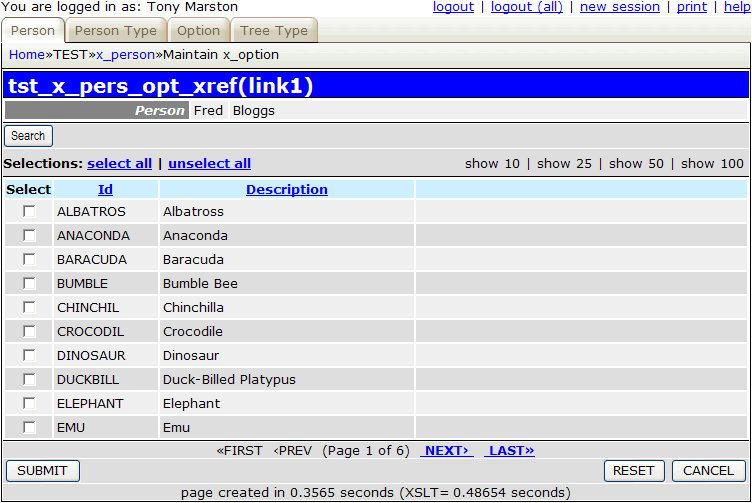### 5. Run the new Transaction

When you run this transaction from the List Person screen it will show the selected X_PERSON entry at the top, with all available X_OPTION entries (split into pages) at the bottom. The checkbox in the 'Select' column will show if a corresponding entry exists on the X_PERS_OPT_XREF table or not.

To add entries to the X_PERS_OPT_XREF table simply set the checkbox from OFF to ON and press the SUBMIT button.

To remove entries from the X_PERS_OPT_XREF table simply set the checkbox from ON to OFF and press the SUBMIT button.

It is possible to filter the details being displayed by pressing the 'Search' button which will bring up the screen shown in Figure 13:

Figure 13 - search X_OPTION screen (original)By entering the required selection criteria and pressing the SUBMIT button control will be returned to the previous screen, and the selection criteria will be used when retrieving data from the database. However, it may be useful to be able to select records on the value of the 'Select' column, as shown in Figure 14:

Figure 14 - search X_OPTION screen (modified)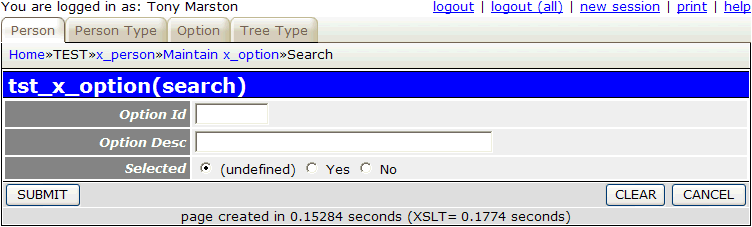By default the 'Selected' column is 'undefined', which means that the value is not included in any selection criteria. If it is set to 'Yes' then only those records where the 'Select' column is checked ON will appear in the display, and if it is set to 'No' then only those records where the 'Select' column is checked OFF will appear in the display. This field can be added to the search screen by following these steps:

First, add the following line to the `x_option.detail.screen.inc` file:

```\$structure['main']['fields'][] = array('selected' => 'Selected');
```

Second, add the following code to the _cm_changeConfig() method in the `x_option.class.inc` file:

```    function _cm_changeConfig (\$where, \$fieldarray)
{
if (\$GLOBALS['mode'] == 'search') {
\$prev_script = getPreviousScript();
\$pattern_id  = getPatternId(\$prev_script);
// add an extra field to the field list
\$this->fieldspec['selected'] = array('type' => 'boolean');
} // if
} // if

return \$fieldarray;

} // _cm_changeConfig
```

This transaction will list X_PERS_OPT_XREF entries for a selected X_PERSON, but it may also be useful to have another screen which lists X_PERS_OPT_XREF entries for a selected X_OPTION. The procedure is exactly the same as that shown above, but with the following variations:

1. On this screen simply set 'Outer table = x_option' and 'Inner table = x_person', then edit the default values for Task Id, Script Id and Screen Structure to change 'link1' to something different, such as 'link1a', otherwise the procedure will fail due to a duplicate value.
2. In this procedure you add the newly generated transaction to the navigation button for parent transaction `tst_x_option(list1)`.
3. In this procedure you make the corresponding modifications to the `x_person.detail.screen.inc` and `x_person.class.inc` files to add the 'Selected' column to the search screen.

### 6. Modify screen labels and button text

Follow the procedure described in Part 2 of this tutorial to replace the default text with customised values.

## References